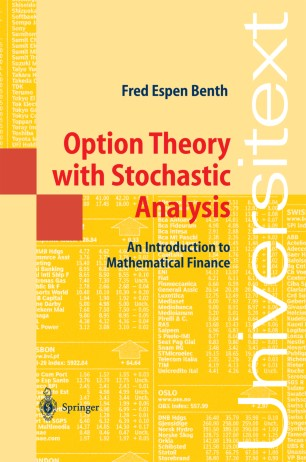# Option Theory with Stochastic Analysis

## An Introduction to Mathematical Finance

• Very concise, requires only basic mathematical skills

• Describes the basic assumptions (empirical finance) underlying option theory

• Includes a big section on pricing using both pde-approach and martingale approach (stochastic finance)

• Presents the two main approaches for numerical computation of option prices (computational finance)

• Can be used at introductory level at universities, with exercises after each chapter

• Potential interest for the German actuaries and actuarial trainingTextbook

Part of the Universitext book series (UTX)

1. Front Matter
Pages I-X
2. Fred Espen Benth
Pages 1-10
3. Fred Espen Benth
Pages 11-32
4. Fred Espen Benth
Pages 33-52
5. Fred Espen Benth
Pages 53-97
6. Fred Espen Benth
Pages 99-119
7. Back Matter
Pages 121-166

### Introduction

The objective of this textbook is to provide a very basic and accessible introduction to option pricing, invoking only a minimum of stochastic analysis. Although short, it covers the theory essential to the statistical modeling of stocks, pricing of derivatives (general contingent claims) with martingale theory, and computational finance including both finite-difference and Monte Carlo methods. The reader is led to an understanding of the assumptions inherent in the Black & Scholes theory, of the main idea behind deriving prices and hedges, and of the use of numerical methods to compute prices for exotic contracts. Finally, incomplete markets are also discussed, with references to different practical/theoretical approaches to pricing problems in such markets.
The author's style is compact and to-the-point, requiring of the reader only basic mathematical skills. In contrast to many books addressed to an audience with greater mathematical experience, it can appeal to many practitioners, e.g. in industry, looking for an introduction to this theory without too much detail.
It dispenses with introductory chapters summarising the theory of stochastic analysis and processes, leading the reader instead through the stochastic calculus needed to perform the basic derivations and understand the basic tools
It focuses on ideas and methods rather than full rigour, while remaining mathematically correct.
The text aims at describing the basic assumptions (empirical finance) behind option theory, something that is very useful for those wanting actually to apply this. Further, it includes a big section on pricing using both the pde-approach and the martingale approach (stochastic finance).
Finally, the reader is presented the two main approaches for numerical computation of option prices (computational finance). In this chapter, Visual Basic code is supplied for all methods, in the form of an add-in for Excel.
The book can be used at an introductory level in Universities. Exercises (with solutions) are added after each chapter.

### Keywords

Analysis Gaussian distribution Measure Normal distribution Option Pricing Options Probability theory Statistical Analysis modeling

#### Authors and affiliations

1. 1.Department of MathematicsCentre of Mathematics for Applications University of OsloOsloNorway

### Bibliographic information

• Book Title Option Theory with Stochastic Analysis
• Book Subtitle An Introduction to Mathematical Finance
• Authors Fred Espen Benth
• Series Title Universitext
• DOI https://doi.org/10.1007/978-3-642-18786-5
• Copyright Information Springer-Verlag Berlin Heidelberg 2004
• Publisher Name Springer, Berlin, Heidelberg
• eBook Packages
• Softcover ISBN 978-3-540-40502-3
• eBook ISBN 978-3-642-18786-5
• Series ISSN 0172-5939
• Edition Number 1
• Number of Pages X, 162
• Number of Illustrations 1 b/w illustrations, 0 illustrations in colour
• Topics
• Buy this book on publisher's site
Industry Sectors
Pharma
Automotive
Chemical Manufacturing